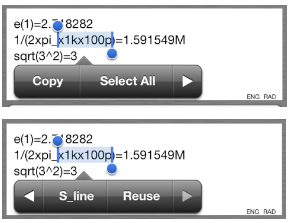# アナログ回路設計コンサルタント　株式会社e-skett

## アナログ電子回路設計に関する技術コンサルティングのご用命は株式会社ｅ－ｓｋｅｔｔ

〒253-0012 茅ヶ崎市小和田三丁目８番-12-611号

How to use e-calcPro

## help

### 1. Features

This calculator can be used not only in scientific notation(e.g. 1E-6) but also using engineering multipliers(e.g. p,n,u,m,k,M).

• Calculations History and reuse it
• Can use engineering multipliers,6 Special buttons
• Four user-defined constant
• Four interactive variable
• Three buttons can be insert formula
• interactive variable can have alias
• Functions: √,log,ln,sin,cos,tan,sinh,cosh,tanh,asin,acos,atan,asinh,acosh,atanh,e^x
• Built-in Constants:π,bk,q,e0 and Operator　^
• Autocomplete parentheses
• ON/OFF function key click sound
• Mail transmission function of the calculation history

### 2.Summary

This is a screen shot of the e-calcPro.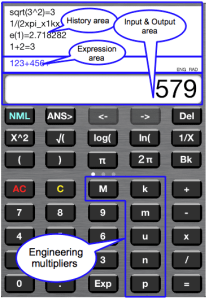Visible part is divided into three.
The bottom is the input and output display area,Similar to the general part of the calculator display.
On it, is the area formula to display the formula in the current calculation.
The top is a history area and to display the result of a calculation formula of the past.
There is a mode display area in the lower right corner of the area formula.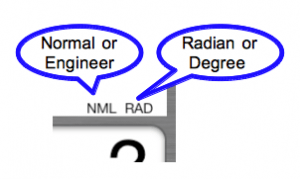You can use an electrical engineer commonly used engineering units in e-calcPro.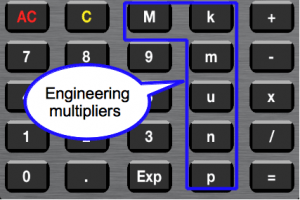Each button meaning,
p=1E-12,n=1E-9,u=1E-6,m=1E-3,k=1E3,M=1E6 .
Can be entered directly from the button is these six, the display is also used f = 1E-15, G = 1E9.
By pressing the  ENG(NML) button , you will be able to choose whether to use the exponential format display, whether to display using this auxiliary unit.
However,  in the case of the result  is less than 1E-16 or even more than 1E15  using the exponential form.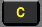is a clear button.s reset to 0 input and output display area.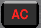is ALL Clear button. Clear input & output area  also Expression area.
Without pressing the AC button, after pressing the equal  button , so that the following calculations can continue.
Please press the AC button to do when things go wrong.
√ When calculating, please enter the order √ button, and then  numeric value.
We will be as follows: Press the button when calculating the √ 3, for example.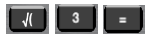not entered the right parenthesis, which is auto-completion.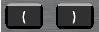Also, if when you press the = button, the number of right parentheses is not enough,  automatically insert the right parenthesis to the right with the necessary number of expressions.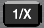When you press this button after entering 1 +2 +3, converted to expression 1 / (1 +2 +3) (only convert,  do not do the  calculation.)
Do not use this button, if you want the 1 +2 +1 / 3 Please enter formulas to reason.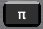This button will insert the word pi_ expression.  pi_ = 3.141593 … is the actual calculation.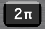This button is only to insert the word 2xpi_ expression. I think in the calculation, such as the cut-off frequency is convenient to use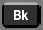This button will insert the word bk_ expression. This is the Boltzmann’s constant. Calculation time is converted to bk_ = 1.3807E-23

### 3.Functions

Another function button will appear when you swipe with your finger to the left part of the function button.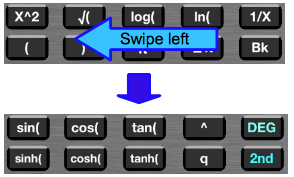In addition, another function button also appears, and then press the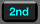button.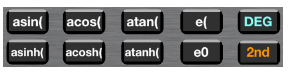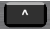This button is the exponentiation operator. We then used to calculate the x ^ y.
can not directly enter the functions and constants after the “^”.
When used as an index of functions and constants, please enter before a “(“.
When you press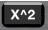button, after the number of the input area, the word ^ 2 is added.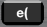button is a function that shows the power of the base of the natural logarithm e. e(3) =e ^ 3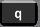button will insert the word q_ expression. This is the elementary charge. Calculation time is converted to q_ = 1.60218E-19.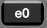button will insert the word e0_ expression. This is the permittivity of vacuum. Calculation time is converted to eo_ = 8.854E-12.
constants that can be used in ecalcPro is as follows.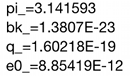### 4.Using the constant

You will be shown below when you swipe to the left to further function area.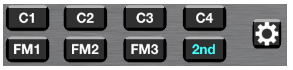C1 ~ C4 is a  user-defined constant buttons. You can be used to store frequently used constants.
aIn addition, FM1 ~ FM4 is the formula button, you will be able to store a formula frequently used, recalling when needed. To put in the formula C5 ~ C6 interactive input variable, which will be described later, it is more convenient to use.
whenbutton is pressed, the screen will be displayed for the registration of the formula and constant.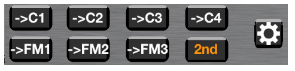enter a number in the input and output area,Constant is stored in the C1_ When you press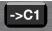button.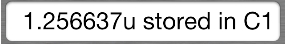This display disappears in 1 second
Press the C1 button to use the constant  was stored. In this case, you can choose to add the word to the formula C1_, or add a number to convert.
Can be set in the setting screen will be described later.

### 5.interactive variable

Similarly, by pressing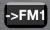button, you will be able to be stored in the FM1 button formula Formula area.
The following sections describe how to use the interactive input variable.
You will be in edit mode, tap the area formula with the 2nd button is pressed, C5 ~ C8 button will appear as shown in the figure below.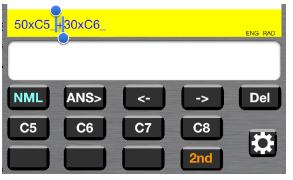We’ve finished entering expressions using this button C5 ~ C8,Please exit from edit mode by tapping the area formula again.

Then, when you press the = button, e-calcPro will ask the value of the variable as follows. Please press the .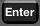button(= button) after entering the number.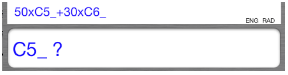It is possible to define an alias in a variable called C5_~ C8_.
Please open the setting screen by pressing this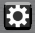button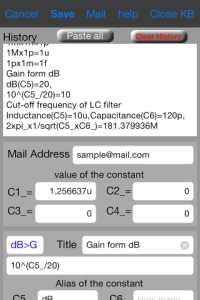Example of setting of FM1 part of this setting screen will be the next screen.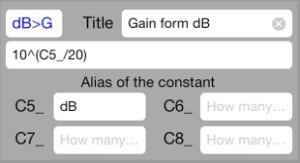Expression that you saved usebutton is on the second line.
Please enter the words you like part of the title of the expression and the alias definition.
It is a character string of blue is displayed on the button.
Then, press the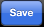button, please return to the calculator screen.

Pushbutton,and  When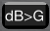button is pressed, the formula that the title of the expression appears in the history area, stored in the area formula will be displayed.Press the = button in that state, waiting to be entered to display the numeric alias you have defined as shown in the figure below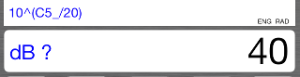e-calcPro can only be used with a fixed variable name. However, the interactive input is possible in this way in an easy-to-understand way.

### 6.email and setup screen

Configuration screen that you can:

• Edit History
• Checking and editing the constant C1 ~ C4
• Edit formulas and of FM1 ~ FM3, alias definitions of the variables
• You can send e-mail, such as the calculation history
• You can paste pasteboard via constant that you saved, the variable alias  etc..
• Switching ON / OFF of the key click sound
• Limit set number of digits displayed to the right of the decimal point in the engineering mode
• How to set the insertion of C1 ~ C4 constant

Including the calculation history contents, this setting is displayed on the screen are automatically saved when you exit the app, re-read at startup.
If you are using long, since a large number of rows of the calculation history, please delete as appropriate. History area is available and can be erased by selecting the part as a normal text editor, or you can append a note. It is also possible to use the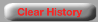button Delete all.

When you press this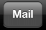button, send mail screen similar to the following is displayed.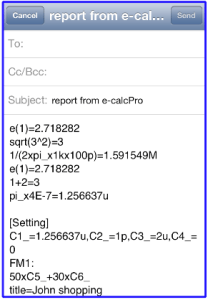In this example, the destination has become empty, if you register frequently used destination address in the email address field of the previous setting screen, it will automatically appear in the destination field of this screen.

Please copy the part of “up to this line” and “from this line.”
Next, return to the setting screen, please press the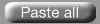button.
And  go back to the calculator screen by pressing the Save button.

from this line
[Setting]
C1_=0.534483,C2_=0,C3_=0,C4_=0
FM1:dB>G
10^(C5_/20)
title=gain from dB
C5_=dB,C6_=,C7_=,C8_=
FM2:G>dB
20xlog(C5_)
title=dB from gain
C5_=gain,C6_=,C7_=,C8_=
FM3:LCfil
1/(2xpi_xsqrt(C5_xC6_))
title=Cut-off frequency of the LC filter
C5_=Inductance,C6_=Capacitance,C7_=,C8_=
up to this line

### 7.Using the other buttons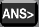This button copies the input and output display area the previous calculation results.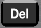This button is used to erase one character at the input and output display area.

Edit mode by tap the area formulas, which will change yellow.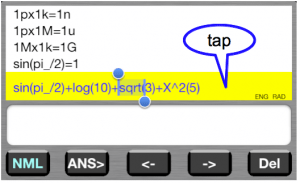This mode can be use cursor button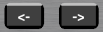,you will be able to move the selected area using the cursor button.
Selected area will be erased when you press thisbutton.
When you press the function button and the calculation button and number button, the character is inserted to the right of the cursor.
Selection area extends to its length part of the functions and constants.
Press the cursor left button when the selected region further reaches the left end, the selected area will disappear, but if you type something at this time, characters will be inserted on the left side.
When you return to the normal mode by tapping the area formula again, you will be able to continue the calculation after editing.
It should be noted that, after even without entering the edit mode, press the = button, by pressing a button operation, if you want to calculate and add the expression to the result of the last, or you can add an expression to the formula of the previous can.

### 8.How to reuse the formula

You can reuse the previous formula.
Please double-tap or press and hold, the expression that you want to re-use history of the area. Part of the expression is selected, a pop-up menu is displayed, press the button “S_line” (Select line) in it, a line is selected.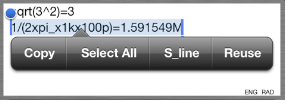Then, when you press the button, “reuse”, part of the formula is copied to the area formula, will be in edit mode. After editing appropriately, exits the edit mode, you can press the = button, you will be recalculated
If the pop-up menu that iOS5 would be folded as shown below, and press this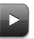button “re-use button” appears.